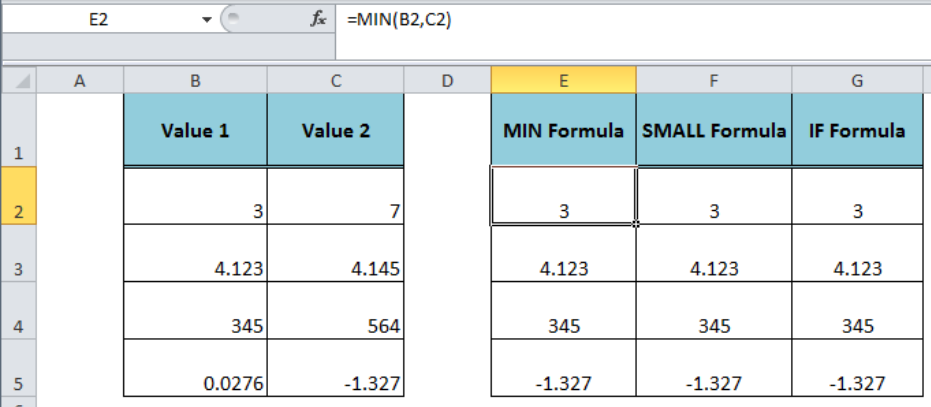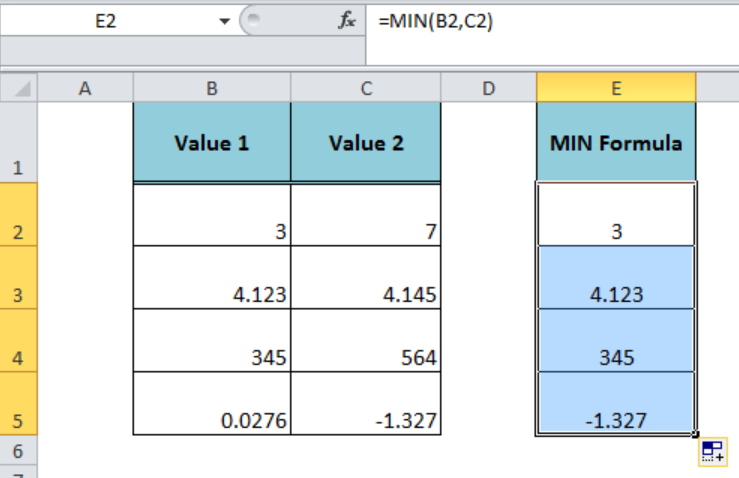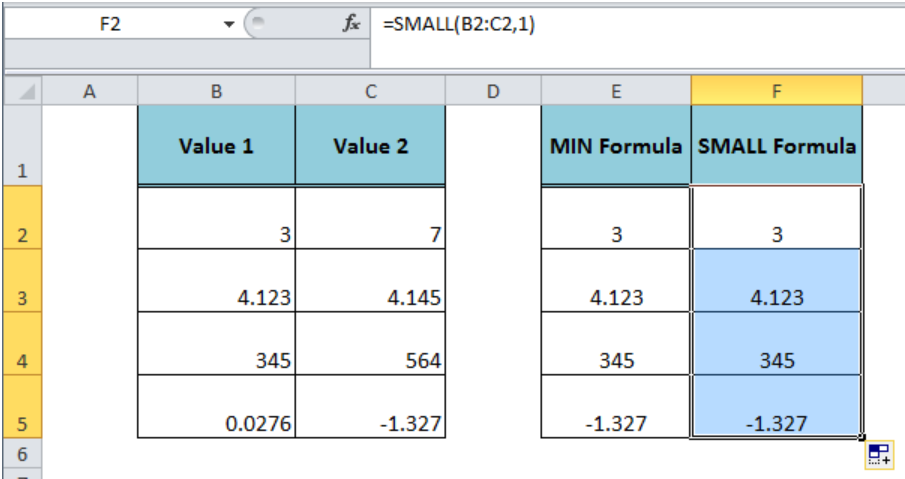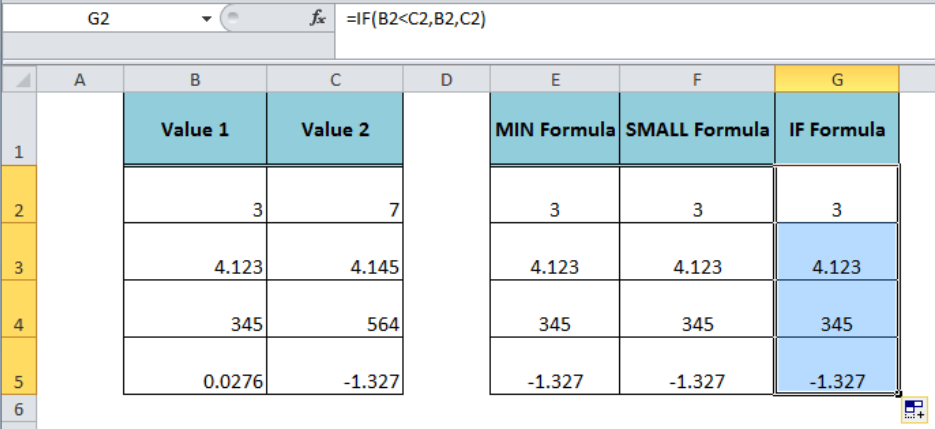Get instant live expert help with Excel or Google Sheets“My Excelchat expert helped me in less than 20 minutes, saving me what would have been 5 hours of work!”

#### Post your problem and you’ll get Expert help in seconds.

Your message must be at least 40 characters
Our professional Expert are available now. Your privacy is guaranteed.

# How to Find the Smaller of Two Values in Excel

When we have numeric values in two cells in Excel and need to compare them to find the smaller of two values, then this can be done using different formulas.Figure 1. Finding the Smaller of Two Values

## Using the MIN Function

The Excel MIN function returns the smallest value in a set of values, ignoring the text and logical values. We can enter the two values in this function as constant numbers, cell reference, range or an array to find the smaller of two values. For our example, we will use the following formula in cell E2 and copy it down to below cells;

`=MIN(B2,C2)`Figure 2. Using the MIN Function

## Using the SMALL Function

The Excel SMALL function returns the k-th smallest value in a dataset. We can enter the values in this function as a range or array argument to return the k-th smallest values. We need to compare the two values in cell F2 to find the smaller of two values in the following formula and copy this formula down;

`=SMALL(B2:C2,1)`Figure 3. Using the SMALL Function

## Using the IF Function

The Excel IF function checks whether a condition is met and returns one value if TRUE, else another value if FALSE. Using the IF function we can compare two values in the logical_test arguments to check the given condition and return one value if TRUE, else the second value. We can find the smaller of two values in the following formula in cell G2 and copy it down;

`=IF(B2<C2,B2,C2)`Figure 4. Using the IF Function

## Instant Connection to an Expert through our Excelchat Service:

Most of the time, the problem you will need to solve will be more complex than a simple application of a formula or function. If you want to save hours of research and frustration, try our live Excelchat service! Our Excel Experts are available 24/7 to answer any Excel question you may have. We guarantee a connection within 30 seconds and a customized solution within 20 minutes.

### Did this post not answer your question? Get a solution from connecting with the expert.Another blog reader asked this question today on Excelchat:
Solution examplesCould you help me by explaining the IF function?
Solved by G. L. in 24 minsI have a question about Conditional Formatting. =IF('Sheet1'!A1="X",IF('Sheet1'!B1="Y", TRUE, FALSE),FALSE) the set the cell background to red. I would like to make it so that if Sheet1'!B1="Z" I set the back to blue. How can I achieve this.
Solved by B. F. in 39 minsI need to find out if the 2018 yearly sales goals were met if the yearly sales were \$25,000 or more using an IF logical function and to set the formula to return a value of YES if met, and NO if not
Solved by A. A. in 18 minsI filtered data in column A (it is labeled ID #) of a data set. On my next worksheet, column A is also the ID #, but it is a different data set. I want to filter the ID #'s the same for the two sheets, but how do I do that being that I am working with two different sets of data?
Solved by V. H. in 22 minsi want to create a very simple if function in a cell which is, if one cell is less than 1250, then the automatic value in the current cell is 250, dead easy, for someone who knows! Thank you.
Solved by A. H. in 14 mins## Subscribe to Excelchat.coAnother blog reader asked this question today on Excelchat: